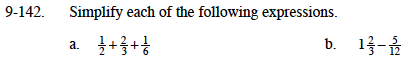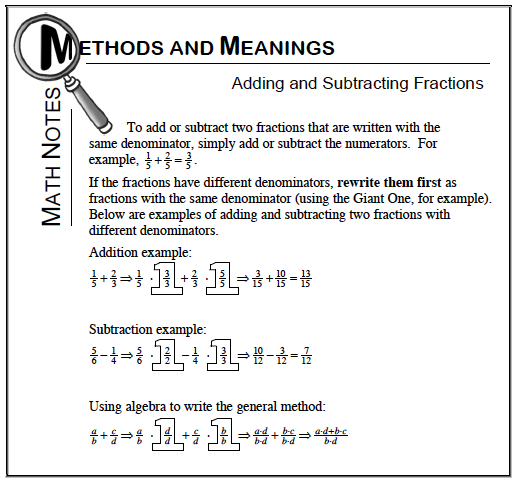### Home > MC1 > Chapter 9 > Lesson 9.3.2 > Problem9-142

9-142.Refer to the Math Note box below for help adding and subtracting fractions.Change each fraction so that the denominator is 6.

$\left(\frac{1}{2}\right)\left(\frac{3}{3}\right)+\left(\frac{2}{3}\right)\left(\frac{2}{2}\right)+\frac{1}{6}=\frac{3}{6}+\frac{4}{6}+\frac{1}{6}$

$\frac{3}{6}+\frac{4}{6}+\frac{1}{6}=\frac{8}{6}=\frac{4}{3}$
$\frac{4}{3}=1\frac{1}{3}$
$\text{Try rewriting }1\frac{2}{3}\text{ as }1\frac{8}{12}.\text{ Does that help you to subtract? How?}$